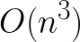## PyOpenGL keyboard won’t respond?

```Below code generates above beautiful image. One problem is keyboard wouldn't respond at all. keyboard() takes a string value when any key is pressed, ESC or q quit the program. All the example codes in PyOpenGL-Demo fail to respond keyboard also. This has been driving me nut!
def keyboard(key, x, y):
# Allow to quit by pressing 'Esc' or 'q'
if ch == chr(27):
sys.exit()
if ch == 'q':
sys.exit()

I checked how Geodome handles keyboard event.  It was identical to PyOpenGL code. glutKeyboardFunc() calls the user defined keyboard function.
glutKeyboardFunc(keyboard)

The keyboard function of Geodome as below takes unsigned char value.
/* change view angle, exit upon ESC */
/* ARGSUSED1 */
static void
key(unsigned char k, int x, int y)
{
switch (k) {
case '2': tesselate_cmd(2, radius) ; break;
case '3': tesselate_cmd(3, radius) ; break;
case '4': tesselate_cmd(4, radius) ; break;
case '5': tesselate_cmd(5, radius) ; break;
case '6': tesselate_cmd(6, radius) ; break;
case '7': tesselate_cmd(7, radius) ; break;
case '8': tesselate_cmd(8, radius) ; break;
case '9': tesselate_cmd(9, radius) ; break;
case 'z': view_roty += 4.0; break;

I inserted print() line that shows key's data type and actual value to debug the problem.
def keyboard(key, x, y):
ch = key.decode("utf-8")
print(type(key), key, type(ch), ch)
# Allow to quit by pressing 'Esc' or 'q'
if ch == chr(27):
sys.exit()
if ch == 'q':
print('Good bye!')
sys.exit()

glutKeyboardFunc() is returning bytes object while keyboard()'s key parameter is expecting string object. So if condition becomes unknown that makes non-responsive to any keyboard event.
```
\$ python3 PyMathArt.py
<class 'bytes'> b'a' <class 'str'> a
<class 'bytes'> b'b' <class 'str'> b
<class 'bytes'> b'c' <class 'str'> c
<class 'bytes'> b'1' <class 'str'> 1
```
Fixed keyboard function
def keyboard(bkey, x, y):
# Convert bytes object to string
key = bkey.decode("utf-8")
# Allow to quit by pressing 'Esc' or 'q'
if key == chr(27):
sys.exit()
if key == 'q':
print("Bye!")
sys.exit()

# python3
# http://www.math.uiuc.edu/~gfrancis/illimath/StanBlank/PyOpenGL.pdf
# Python Programming in OpenGL by Stan Blank, p100
# 4/26/2016
# PyMathArt.py

from OpenGL.GLUT import *       # GL Utilities Toolkit
from OpenGL.GL import *
from OpenGL.GLU import *
import numpy as np
import random
import sys

# Set the width and height of the window with global variables
# Set the axis range globally
global width
global height
global axrng
global stepsize

# Initial values
width = 500
height = 500
axrng = 10.0
stepsize = (2.0 * axrng) / width

return np.pi/180.0 * x

def init():
# red, green, blue, alpha from 0.0 to 1.0
glClearColor(1.0, 1.0, 1.0, 1.0)

# (x-left, x-right, y-bottom, y-top) set the coordinate system ranges
# -1.0 to 1.0 for both x and y, origin(0,0) in the center
# gluOrtho2D(-5.0, 5.0, -5.0, 5.0)

def plotmathart():
glClear(GL_COLOR_BUFFER_BIT)
glBegin(GL_POINTS)

for x in np.arange(-axrng, axrng, stepsize): # 0.04
for y in np.arange(-axrng, axrng, stepsize):
r = np.cos(x) + np.sin(y)
glColor3f(np.cos(y*r), np.cos(x*y*r), np.sin(r*x))
glVertex2f(x, y)
glEnd()
glFlush()

# Keep the aspect ratio of the graphics window and
# anything we draw will look in proper proportion
# OpenGL set w and h values as the graphics window's size changes
def reshape(w, h):

# To insure we don't have a zero widow height
if h == 0:
h = 1
# Fill the entire graphic window
glViewport(0, 0, w, h)

# Set the projection matrix or the world view
glMatrixMode(GL_PROJECTION)

# Set the aspect ratio of the plot
if w <= h:
gluOrtho2D(-axrng, axrng, -axrng*h/w, axrng*h/w)
else:
# gluOrtho2D(-axrng*w/h, axrng*w/h, -axrng, axrng)
gluOrtho2D(-axrng*h/w, axrng*h/w, -axrng, axrng)

# Set the matrix for the object we are drawing
glMatrixMode(GL_MODELVIEW)

def keyboard(key, x, y):
# Allow to quit by pressing 'Esc' or 'q'
if ch == chr(27):
sys.exit()
if ch == 'q':
sys.exit()

"""
Since we declared the reshape function as the glutReshapeFunc() in def main():
, when the pypolar.py program runs, OpenGL/GLUT assumes that the creatio n of the graphics window constitutes a “reshaping” of the window and the reshape function is automatically called or triggered by GLUT.
"""
def main():
global width
global height

glutInit(sys.argv)
glutInitDisplayMode(GLUT_SINGLE | GLUT_RGB)
glutInitWindowPosition(100, 100)
glutInitWindowSize(width, height)
glutCreateWindow("Math Art Patterns")
glutReshapeFunc(reshape)
glutDisplayFunc(plotmathart)
glutKeyboardFunc(keyboard)

init()
glutMainLoop()

main()

Related
```Email: k2.mountain [at] gmail [dot] com Every content on the blog is made by Free and Open Source Software in GNU/Linux.
This entry was posted in GNU/Linux and tagged , , , , , . Bookmark the permalink.

### 4 Responses to PyOpenGL keyboard won’t respond?

1.Akash says:

Thank you very much. You Beautifully pointed out the problem with key variable. This allowed me to crack the issue and go ahead with my old and rusty repository.
Again, Many Thanks,

2.pepe says:

can you clearly tell me the fix? I have the same problem.

•janpenguin says:

Use keyboard(bkey, x, y) function that converts bytes object to string. It will work.

3.Tony Power says:

def keyboard( key, x, y ):
if ch == chr(27):
sys.exit()
if ch == ‘q’:
sys.exit()

you can do:

def keyboard( key, x, y ):
if key == b’\x1b’ or key==b”q”:
sys.exit()

Note the b preceding the key value.